# 自然语言深度建模

## 综述序列模型，建模自然语言的核心问题

• 序列建模
• 循环神经网络
• 表达式
• 计算图
• LSTM
• GRU
• 卷积与时间卷积网络
• 全卷积与因果卷积
• 空洞卷积
• 高速公路网络与残差模块
• 实现
• LSTM的语言建模
• 时间卷积网络的语言建模

### 循环网络

#### 表达式

$s ^ { ( t ) } = f \left( s ^ { ( t - 1) } ; \theta \right)$

$s ^ { ( 3) } = f \left( s ^ { ( 2) } ; \theta \right) = f \left( f \left( s ^ { ( 1) } ; \theta \right) ; \theta \right)$

$h ^ { ( t ) } = f \left( h ^ { ( t - 1) } ,x ^ { ( t ) } ; \theta \right)$

$f ( x ) = f ^ { ( 3) } \left( f ^ { ( 2) } \left( f ^ { ( 1) } ( x ) \right) \right)$

#### 计算图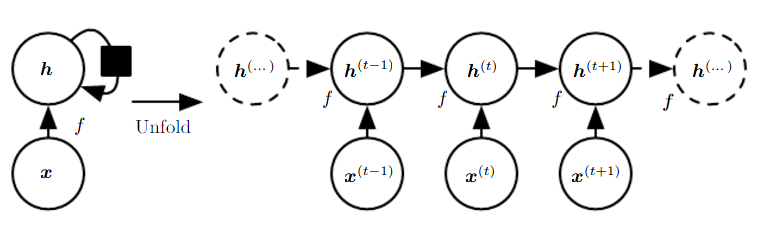Elman Network 代表了一类循环网络，它的每一个时间步都有一个输入与输出，且循环连接发生在隐藏单元与隐藏单元之间。我们通过累积每一个预测 $\hat{y}$ 与 $y$ 之间的误差来确定损失函数 L，并执行沿时间的反向传播训练整个网络。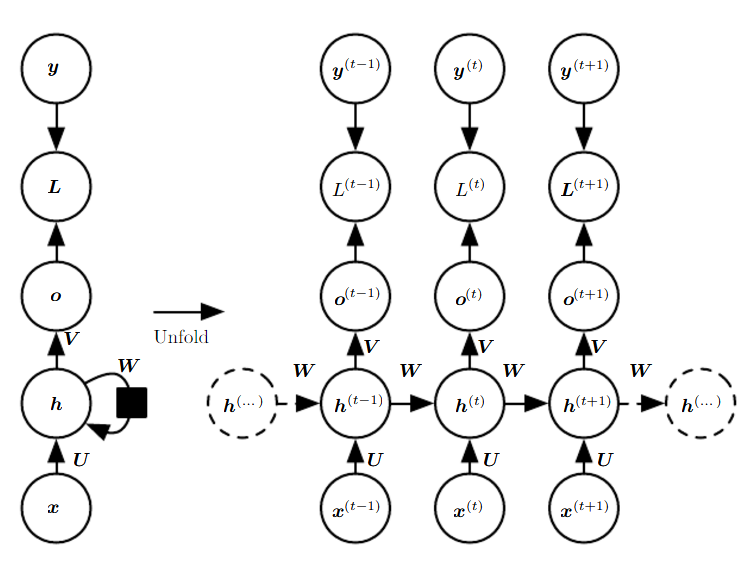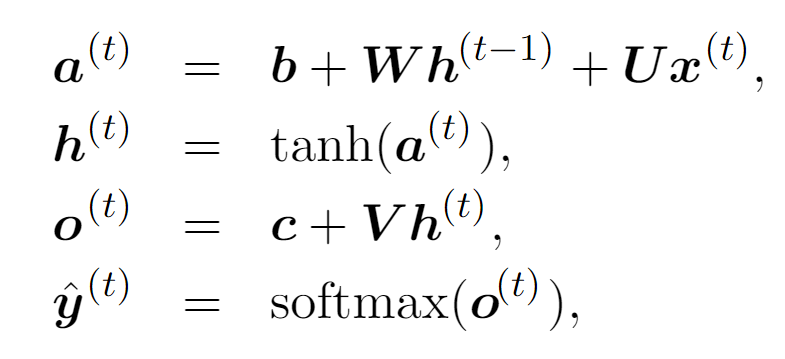Jordan Network 代表了一类循环网络，它的每一个时间步都有一个输入与输出，但循环连接只存在当前时间步的输出和下一个时间步的隐藏单元之间。因为该架构在隐藏单元之间没有循环连接，因此它没有一个记忆机制来捕捉所有用于预测未来时间步的历史信息。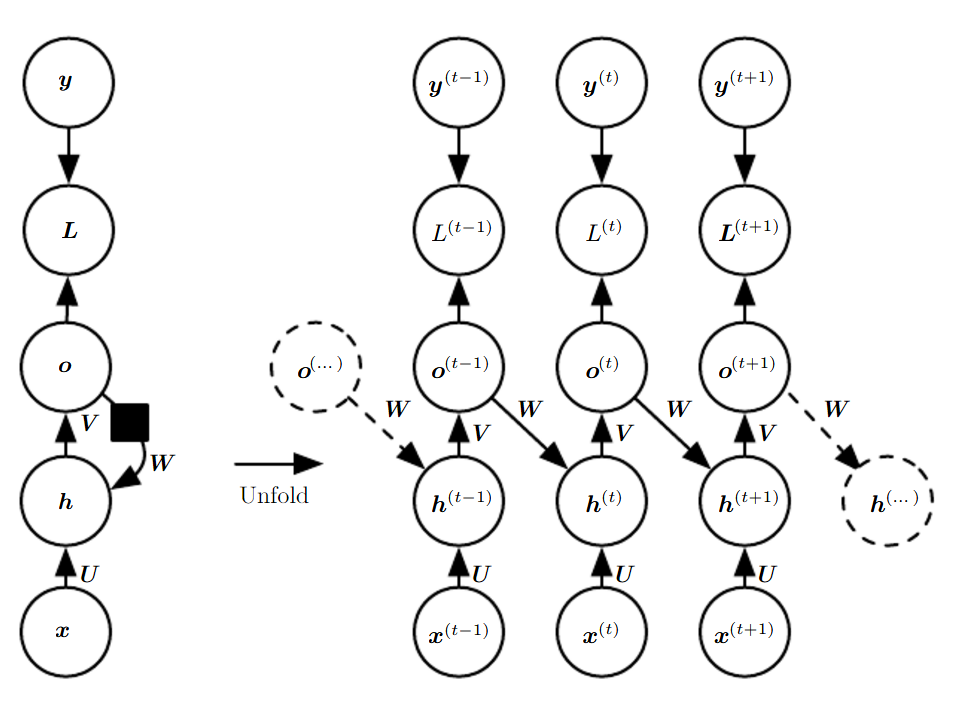Jordan Network 类的架构在推断时还是会使用前一时间步的输出值 o 来计算后一时间步的隐藏状态。这种网络的一大缺点是，训练过程中观察到的数据与测试时看到的数据会有较大的不同。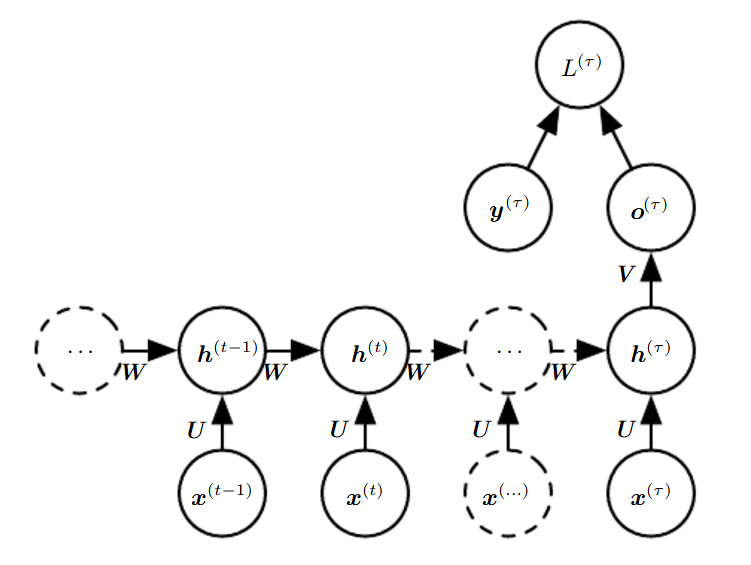#### LSTM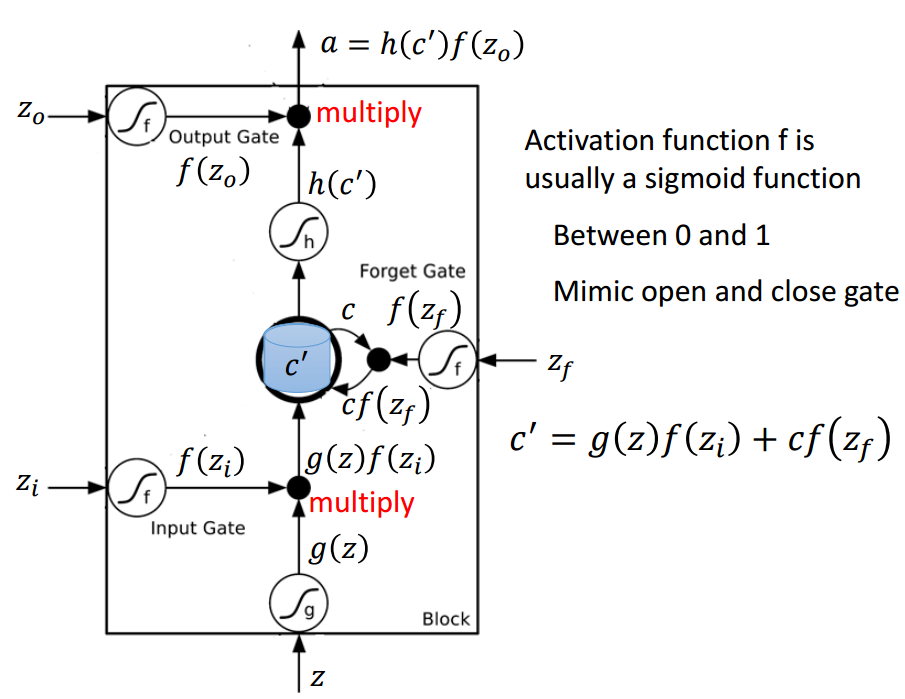### 实现

#### LSTM 语言建模

TCN 语言建模